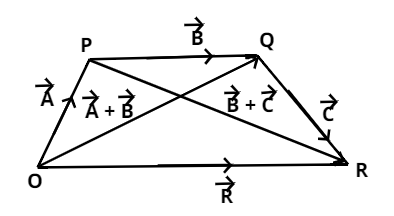Courses
Courses for Kids
Free study material
Offline Centres
MoreLast updated date: 05th Dec 2023
Total views: 382.5k
Views today: 6.82k

# Write the associative law of addition of vectors.Verified
382.5k+ views
Hint: Let us prove the associative law of addition of vectors by using a parallelogram with its sides as different vectors.Now as we know that the associative law of addition of vectors states that the sum of the vectors remains same irrespective of their order or grouping in which they are arranged.
Like if $\overrightarrow {\text{X}}$, $\overrightarrow {\text{Y}}$ and $\overrightarrow {\text{Z}}$ are the three vectors then according to associative law of addition of vectors $\left( {\overrightarrow {\text{X}} {\text{ + }}\overrightarrow {\text{Y}} } \right){\text{ + }}\overrightarrow {\text{Z}} {\text{ = }}\overrightarrow {\text{X}} {\text{ + }}\left( {\overrightarrow {\text{Y}} {\text{ + }}\overrightarrow {\text{Z}} } \right)$
As we know that according to head to tail rule if the head of one vector joins with the tail of another vector then the sum of both vectors will be the vector formed by the joining tail of one vector with the head of another.
So, now let $\overrightarrow {\text{A}}$, $\overrightarrow {\text{B}}$ and $\overrightarrow {\text{C}}$ are the three vectors then applying head to tail rule to obtain the resultant of $\overrightarrow {\text{A}} {\text{ + }}\overrightarrow {\text{B}}$ and $\overrightarrow {\text{B}} {\text{ + }}\overrightarrow {\text{C}}$
So, $\overrightarrow {\text{A}} {\text{ + }}\overrightarrow {\text{B}}$ = $\overrightarrow {{\text{OQ}}}$
And, $\overrightarrow {\text{B}} {\text{ + }}\overrightarrow {\text{C}}$ = $\overrightarrow {{\text{PR}}}$
Then finally again find the resultant of these three vectors,
So, $\overrightarrow {{\text{OR}}} {\text{ = }}\overrightarrow {{\text{OP}}} {\text{ + }}\overrightarrow {{\text{PR}}}$
$\overrightarrow {\text{R}} {\text{ = }}\overrightarrow {\text{A}} {\text{ + }}\left( {\overrightarrow {\text{B}} {\text{ + }}\overrightarrow {\text{C}} } \right)$ (1)
And, $\overrightarrow {{\text{OR}}} {\text{ = }}\overrightarrow {{\text{OQ}}} {\text{ + }}\overrightarrow {{\text{QR}}}$
$\overrightarrow {\text{R}} {\text{ = }}\left( {\overrightarrow {\text{A}} {\text{ + }}\overrightarrow {\text{B}} } \right){\text{ + }}\overrightarrow {\text{C}} {\text{ }}$ (2)
$\left( {\overrightarrow {\text{A}} {\text{ + }}\overrightarrow {\text{B}} } \right){\text{ + }}\overrightarrow {\text{C}} {\text{ = }}\overrightarrow {\text{A}} {\text{ + }}\left( {\overrightarrow {\text{B}} {\text{ + }}\overrightarrow {\text{C}} } \right)$